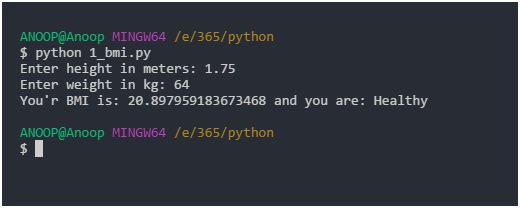# BMI (Body Mass Index) calculator in Python

In this Python article, we are going to learn how to create a BMI (stands for - Body Mass Index) calculator?
Submitted by Anoop Nair, on November 09, 2017

Given weight and height of a person and we have to find the BMI (Body Mass Index) using Python.

Example:

```    Input:
Height = 1.75
Weigth = 64

Output:
BMI is: 20.89 and you are: Healthy
```

The steps that we will follow are:

• We will first get input values from user using input() and convert it to float using float().
• We will use the BMI formula, which is weight/(height**2).
• Then print the result using conditional statements.
• Here we have used elif because once we satisfy a condition we don’t want to check the rest of the statements.

## Program to calculate BMI in Python

```# getting input from the user and assigning it to user

height = float(input("Enter height in meters: "))
weight = float(input("Enter weight in kg: "))

# the formula for calculating bmi

bmi = weight/(height**2)
# ** is the power of operator i.e height*height in this case

print("Your BMI is: {0} and you are: ".format(bmi), end='')

#conditions
if ( bmi < 16):
print("severely underweight")

elif ( bmi >= 16 and bmi < 18.5):
print("underweight")

elif ( bmi >= 18.5 and bmi < 25):
print("Healthy")

elif ( bmi >= 25 and bmi < 30):
print("overweight")

elif ( bmi >=30):
print("severely overweight")
```

OutputIf you liked the article or have any doubt, please write in the comment box.

Preparation